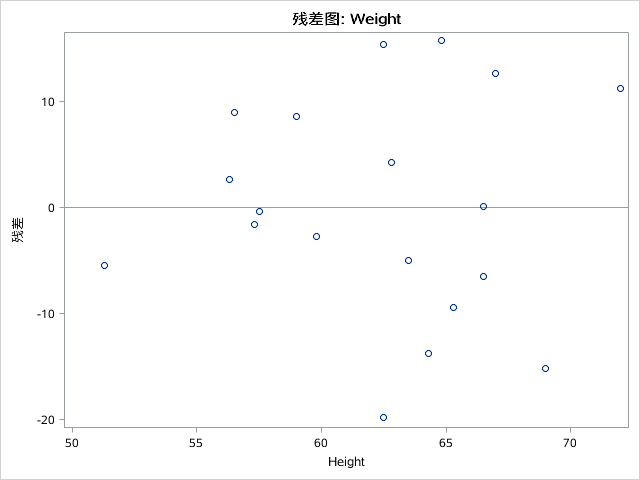## PROC GLM Residuals by Regressor Plot

PROC REG produces a Residuals by Regressors plot. In the past, using PROC REG, I have used this plot to verify that the residuals are normally distributed with a mean of 0.

I am trying to produce this same plot along w/ the other plots in the DIAGNOSTICS option using PROC GLM. Neither of the 2 programming statements below produce this particular plot. Any suggestions?

PROC GLM DATA=want plots(only)=diagnostics(unpack);

PROC GLM DATA=want PLOTS(UNPACK)=DIAGNOSTICS;

1 ACCEPTED SOLUTION

Accepted SolutionsKsharp
Super User

## Re: PROC GLM Residuals by Regressor Plot

Did you try PLOTS=ALL ?

``````proc glm data=sashelp.class plots=all;
class sex;
model weight=sex height/solution;
run;``````Ksharp
Super User

## Re: PROC GLM Residuals by Regressor Plot

Did you try PLOTS=ALL ?

``````proc glm data=sashelp.class plots=all;
class sex;
model weight=sex height/solution;
run;``````Discussion stats